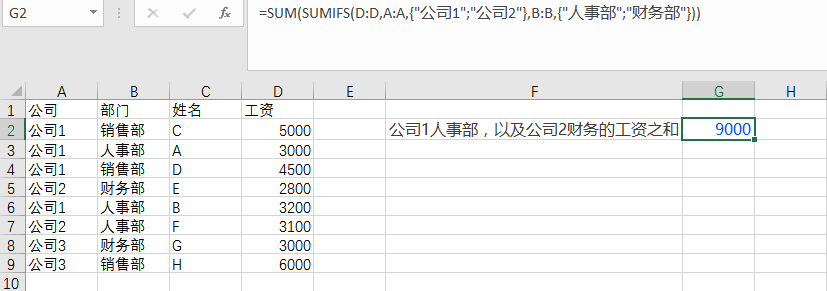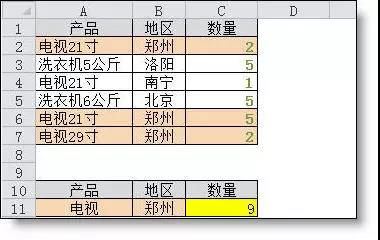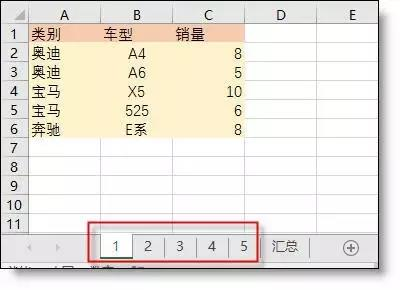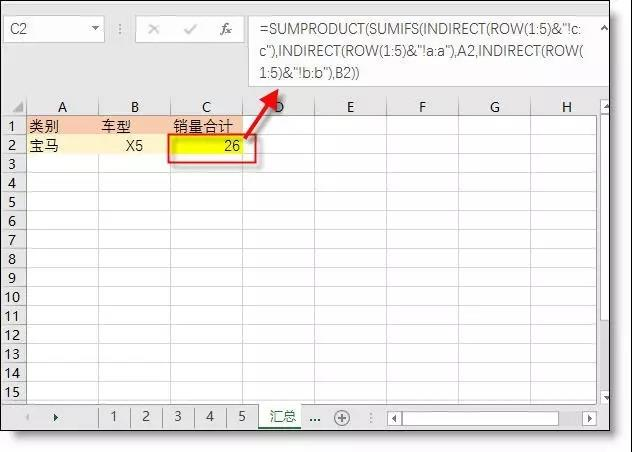# 5个Sumifs函数经典公式用法

1、多条件（并列）求和

=SUMIFS(D:D,A:A,"公司1",B:B,"人事部")2、 多条件（或者）求和

=SUM(SUMIFS(D:D,A:A,{"公司1";"公司2"},B:B,{"人事部";"财务部"}))3、按时间段求和

=SUMIFS(C:C,A:A,">="&F2,A:A,"<="&F3,B:B,F4)4、模糊条件求和

=SUMIFS(C2:C7,A2:A7,A11&"*",B2:B7,B11)5、多表多条件求和
1~5号的车辆销售明细表=SUMPRODUCT(SUMIFS(INDIRECT(ROW(1:5)&"!c:c"),INDIRECT(ROW(1:5)&"!a:a"),A2,INDIRECT(ROW(1:5)&"!b:b"),B2))